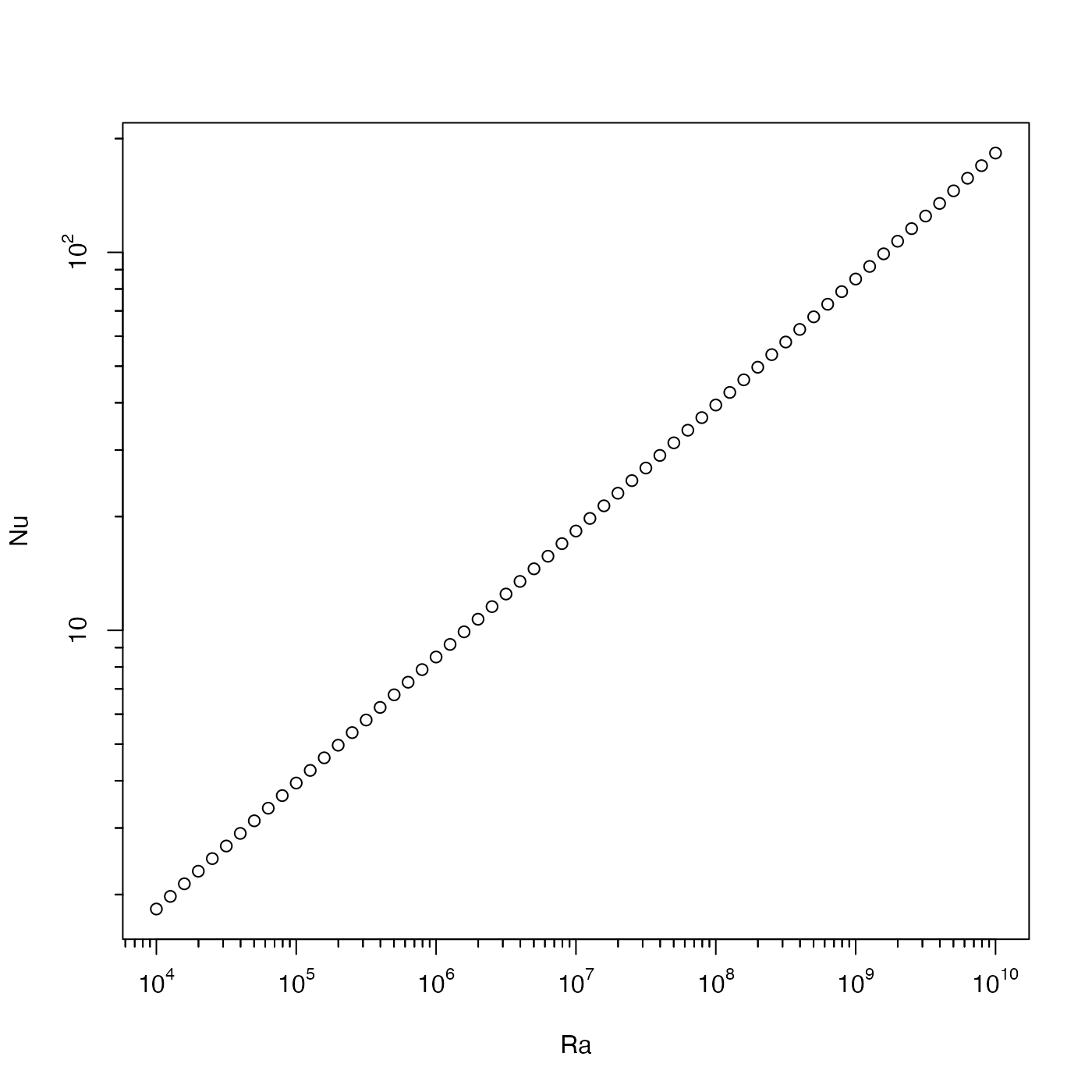Draw an axis, possibly with decade-style logarithmic scaling

oceAxis(side, labels = TRUE, logStyle = "r", ...)

## Arguments

side an integer specifying which axis to draw, with 1 for bottom axis, 2 for left axis, 3 for top axis, and 4 for right axis (as with axis()). either a vector of character values used for labels or a logical value indicating whether to draw such labels. The first form only works if the coordinate is not logarithmic, and if logStyle is "r". a character value that indicates how to draw the y axis, if log="y". If it is "r" (the default) then the conventional R style is used, in which a logarithmic transform connects y values to position on the "page" of the plot device, so that tics will be nonlinearly spaced, but not organized by integral powers of 10. However, if it is "decade", then the style will be that used in the scientific literature, in which large tick marks are used for integral powers of 10, with smaller tick marks at integral multiples of those powers, and with labels that use exponential format for values above 100 or below 0.01. other graphical parameters, passed to axis().

## Value

Numerical values at which tick marks were drawn (or would have been drawn, if labels specified to draw them).

## Examples

library(oce)
Ra <- 10^seq(4, 10, 0.1)
Nu <- 0.085 * Ra^(1/3)
plot(Ra, Nu, log="xy", axes=FALSE)box()oceAxis(1, logStyle="decade")oceAxis(2, logStyle="decade")